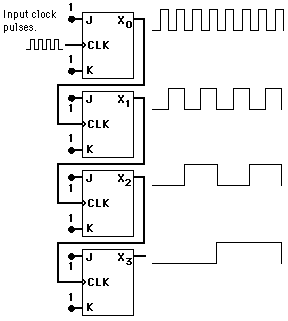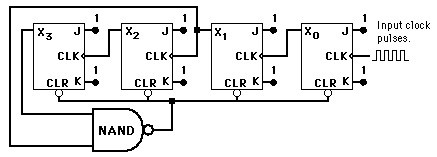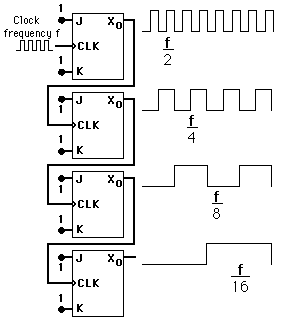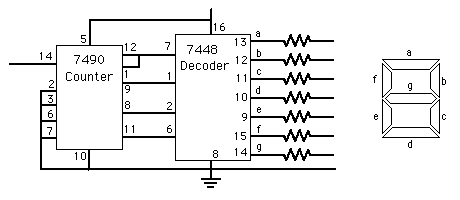# Binary Counting

A binary counter can be constructed from J-K flip-flops by taking the output of one cell to the clock input of the next. The J and K inputs of each flip-flop are set to 1 to produce a toggle at each cycle of the clock input. For each two toggles of the first cell, a toggle is produced in the second cell, and so on down to the fourth cell. This produces a binary number equal to the number of cycles of the input clock signal. This device is sometimes called a "ripple through" counter. The same device is useful as a frequency divider.Index

Electronics concepts

Digital Circuits

Reference
Tocci
Digital Systems, Sec 5-19.

 HyperPhysics*****Electricity and magnetism R Nave
Go BackA BCD counter or decade counter can be constructed from a staight binary counter by terminating the "ripple-through" counting when the count reaches decimal 9 (binary 1001). Since the next toggle would set the two most significant bits, a NAND gate tied from those two outputs to the asynchronous clear line will start the count over after 9.

Index

Electronics concepts

Digital Circuits

Reference
Tocci
Digital Systems, Sec 7-2.

 HyperPhysics*****Electricity and magnetism R Nave
Go Back

# Frequency Division

A frequency divider can be constructed from J-K flip-flops by taking the output of one cell to the clock input of the next. The J and K inputs of each flip-flop are set to 1 to produce a toggle at each cycle of the clock input. For each two toggles of the first cell, a toggle is produced in the second cell, so its output is at half the frequency of the first. The output of the fourth cell is 1/16 the clock frequency. The same device is useful as a binary counter.Index

Electronics concepts

Digital Circuits

Reference
Tocci
Digital Systems, Sec 5-19.

 HyperPhysics*****Electricity and magnetism R Nave
Go Back

# Counter and Decoder

The 14-pin 7490 counter chip and the 16-pin decoder chip are often used together to drive 7-segment displays.Index

Electronics concepts

Digital Circuits

Reference
Mims, Getting started...
p. 90,117

 HyperPhysics*****Electricity and magnetism R Nave
Go Back